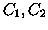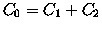Next: 3.1 Bad conditioning Up: THE ASTEROID IDENTIFICATION PROBLEM Previous: 2.3 Restricted orbit identification

# 3. Problems with the linear theory

The linear theory for orbit identification provides the most rigorous mathematical setting to solve this problem. A mathematical theorem, however, is not at all a rigorous tool unless all the hypothesis are applicable to the concrete problem to be solved. The hypothesis needed to apply the formalism of Section 2 are the following:

1.
The normal matricesandare invertible and positive-definite.
2.
The map between the space of the residualsand the space of estimated parametersis in the linear regime, that is the linearized mapis a good approximation in a region including both orbits.
3.
The observation errors are of a size consistent with the residual normalization adopted.

In this Section we shall discuss the applicability of these three hypothesis to the problem of orbit identification.

Maria Eugenia Sansaturio
1999-05-20